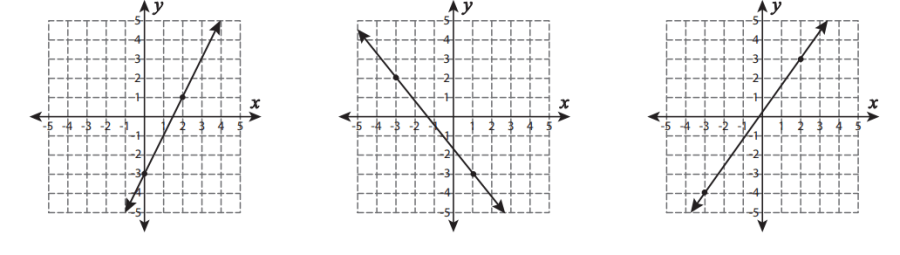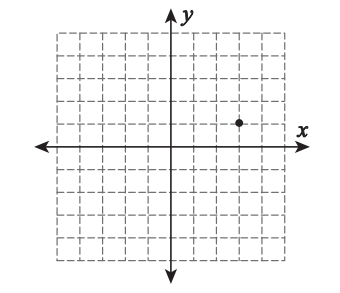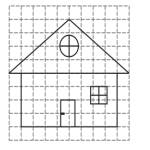# Worksheets## Identify the types of slope

Identify the negative, positive, zero, and undefined slopes of the line. Identify the types of slope on the basis of the position of the graph's lines.## Draw lines on a graph using points: Types of slope

In this part, you have to plot the given points on the graph, draw the line by joining the points, and identify the type of slope.## Calculate the rise and run

In this section, calculate the rise and run by using the coordinate points and lines of the slope.## Draw lines on graph

Draw the lines on the graph by using the points plotted on a graph based on the slope of line.## Slope of the roof

In this section, find the slope of the roof and the result must be in the form of a positive slope. These are the fun activity worksheets.